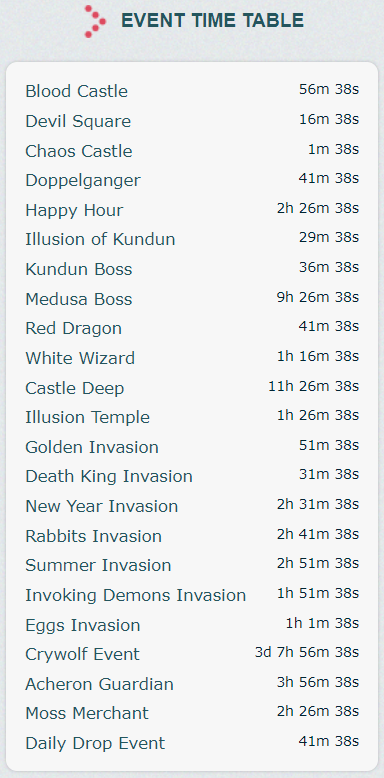Starred Mu

## Recommended Posts

Boss Respawn Times

The time when these bosses will respawn is not known since it depends when someone killed it.

Other respawn times of bosses and events you can check on website Event Time Table.

Server x30 respawns

• Selupan (Raklion) = 2 hours
• Lord Silvester (Urk Mountains) = 12 hours
• Core Magriffy (Nars) = 12 hours
• Lord of Ferea (Ferea) = 12 - 16 hours (random)
• Nix (Nixies Lake) = 12 - 16 hours (random)
• Erohim (Castle Deep, Land of Trials) = 6 hours
• Illusion of Kundun (Kalima 1) = 2 hours
• Illusion of Kundun (Kalima 2) = 4 hours
• Illusion of Kundun (Kalima 3) = 6 hours
• Illusion of Kundun (Kalima 4) = 8 hours
• Illusion of Kundun (Kalima 5) = 10 hours
• Illusion of Kundun (Kalima 6) = 4 hours (see Event Time Table on website)

Server x500 respawns

• Selupan (Raklion) = 2 hours
• Lord Silvester (Urk Mountains) = 6 hours
• Core Magriffy (Nars) = 12 hours
• Lord of Ferea (Ferea)= 6 - 8 hours (random)
• Nix (Nixies Lake) = 6 - 8 hours (random)
• Erohim (Castle Deep, Land of Trials) = 4 hours
• Illusion of Kundun (Kalima 1) = 2 hours
• Illusion of Kundun (Kalima 2) = 4 hours
• Illusion of Kundun (Kalima 3) = 6 hours
• Illusion of Kundun (Kalima 4) = 8 hours
• Illusion of Kundun (Kalima 5) = 10 hours
• Illusion of Kundun (Kalima 6) = 4 hours (see Event Time Table on website)

Server x9999 respawns

• Selupan (Raklion) = 1 hour
• Lord Silvester (Urk Mountains) = 4 hours
• Core Magriffy (Nars) = 6 hours
• Lord of Ferea (Ferea)= 3 - 5 hours (random)
• Nix (Nixies Lake) = 3 - 5 hours (random)
• Erohim (Castle Deep, Land of Trials) = 3 hours
• Illusion of Kundun (Kalima 1) = 1 hours
• Illusion of Kundun (Kalima 2) = 1 hours
• Illusion of Kundun (Kalima 3) = 2 hours
• Illusion of Kundun (Kalima 4) = 2 hours
• Illusion of Kundun (Kalima 5) = 3 hours
• Illusion of Kundun (Kalima 6) = 4 hours (see Event Time Table on website)

Other Boss, Event, Invasions time find on Website "Event Time Table"If you have any suggestion so feel free to leave it in a comment

##### Share on other sites

No info about Kundun ir Land of Trial!

##### Share on other sites
14 minutes ago, Jauniba said:

No info about Kundun ir Land of Trial!

same as normal.

•1

##### Share on other sites

can you add Selupan (Raklion) timer ?

•1